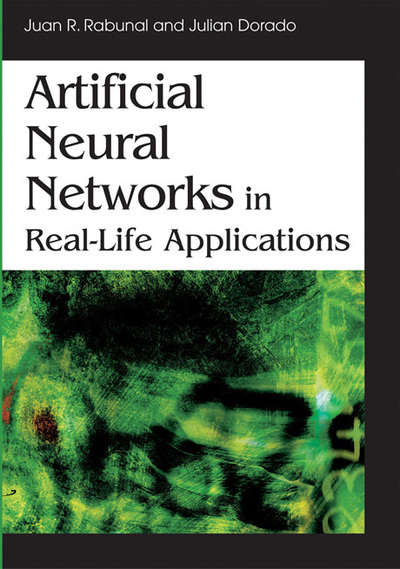# A Neural Network Approach to Cost Minimizatin in a Production Scheduling Setting

Kun-Chang Lee (Sungkyunkwan University, Korea) and Tae-Young Paik (Sungkyunkwan University, Korea)
DOI: 10.4018/978-1-59140-902-1.ch014
Available
\$33.75
List Price: \$37.50
10% Discount:-\$3.75
TOTAL SAVINGS: \$3.75

## Abstract

Cost managers working in manufacturing firms have suffered from the difficulty of determining an optimal cost control strategy. Though the concept of ABC can provide a theoretically nice scheme for cost control, it has been widely known that cost managers have serious trouble comprehending the ABC scheme and applying it to real cost control situations. In this sense, proposing a heuristic method by which cost managers can obtain an approximate cost control strategy comparable to one obtained by ABC would be very meaningful from the view of both theory and practice. To fulfill this need, we suggest using a multi-layered perceptron (MLP) neural network model with backpropagation learning algorithm, and then approximating the optimal cost control strategy of ABC. The primary concern of this study is to investigate whether such solutions approximated by the MLP would be valid from a statistical perspective. If true, it would mean that cost managers can depend on the neural network method to come up with an optimal cost control strategy comparable to applying ABC. To show the validity of the proposed cost control strategy by using the MLP, this study proposes to solve two problems within the context of a production scheduling situation, using ABC: (1) neural network-based total cost estimation (NNTCE); and (2) neural network-based cycle time estimation (NNCTE). For experimental setup, we assume that two products sharing five types of exogenous variables and three types of endogenous variables are manufactured at the same facility. The MLP neural network approach to NNTCE and NNCTE was generated with a set of 180 training data and 125 test data, all of which were proved to be statistically identical with the ABC results.

## Complete Chapter List

Search this Book:
Reset